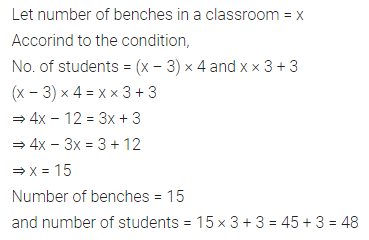ML Aggarwal Class 7 Solutions Chapter 9 Linear Equations and Inequalities Objective Type Questions for ICSE Understanding Mathematics acts as the best resource during your learning and helps you score well in your exams.

## ML Aggarwal Class 7 Solutions for ICSE Maths Chapter 9 Linear Equations and Inequalities Objective Type Questions

Mental Maths

Question 1.
Fill in the blanks:
(i) A linear equation in one variable cannot have more than ………… solution.
(ii) If five times a number is 50, then the number is ……….
(iii) The number 4 is the ………. of the equation 2y – 5 = 3.
(iv) The equation for the statement ‘5 less than thrice a number x is 7’ is ……….
(v) …………. is a solution of the equation 4x + 9 = 5.
(vi) If 3x + 7 = 1, then the value of 5x + 13 is ………..
(vii) In natural numbers, 4x + 5 = -7 has ……….. solution.
(viii) In integers, 3x – 1 = 4 has …………. solution.
(ix) 5x + ………. = 13 has the solution -3.
(x) If a number is increased by 15, it becomes 50. Then the number is ……..
(xi) If 63 exceed another number by 21, then the other number is …………
(xii) If x ∈ W, then the solution set of x < 2 is ………..
Solution: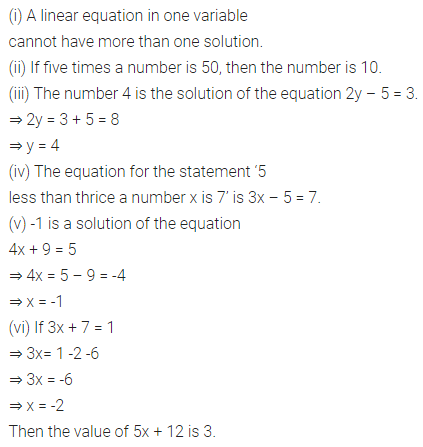Question 2.
State whether the following statements are true (T) or false (F):
(i) We can add (or subtract) the same number of expression to both sides of an equation.
(ii) We can divide both sides of an equation by the same non-zero number.
(iii) 3x – 5 = 2(x + 3) + 7 is a linear equation in one variable.
(iv) The solution of the equation 3(x – 4) = 30 is x = 6.
(v) The solution of the equation 3x – 5 = 2 is x = $$\frac { 7 }{ 3 }$$
(vi) The solution of a linear equation in one variable is always an integer.
(vii) 4x + 5 < 65 is not an equation.
(viii) 2x + 1 = 7 and 3x – 5 = 4 have the same solution.
(ix) $$\frac { 9 }{ 4 }$$ is a solution of the equation 5x – 1 = 8.
(x) If 5 is a solution of variable x in the equation $$\frac { 5x-7 }{ 2 }$$ = y, then the value of y is 18.
(xi) One-fourth of a number added to itself given 10, can be represented as $$\frac { x }{ 4 }$$ + 10 = x.
Solution: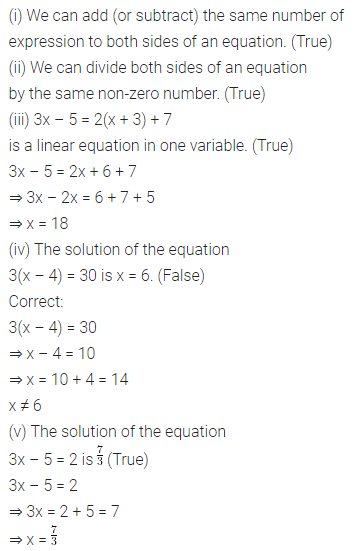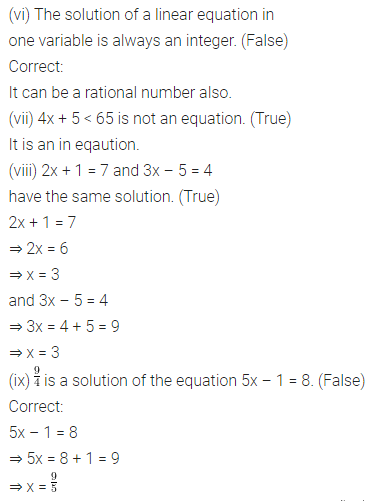Multiple Choice Questions

Choose the correct answer from the given four options (3 to 17):
Question 3.
Which of the following is not a linear equation in one variable?
(a) 3x – 1 = 7
(b) 5y – 2 = 3 (y + 2)
(c) 2x – 3 = $$\frac { 7 }{ 2 }$$
(d) 7p + q = 3
Solution: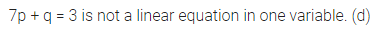Question 4.
The solution of the equation $$\frac { 1 }{ 3 }$$(2y – 1) = 3 is
(a) 5
(b) 3
(c) 2
(d) 1
Solution:Question 5.
x = -1 is a solution of the equation
(a) x – 5 = 6
(b) 2x + 5 = 7
(c) 2(x – 2) + 6 = 0
(d) 3x + 5 = 4
Solution: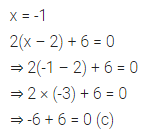Question 6.
If 3(3n – 10) = 2n + 5, then the value of n is
(a) 12
(b) 5
(c) 3
(d) -5
Solution: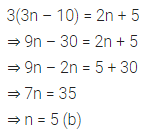Question 7.
-1 is not a solution of the equation
(a) x + 1 = 0
(b) 3x + 4 = 1
(c) 5x + 7 = 2
(d) x – 1 = 2
Solution: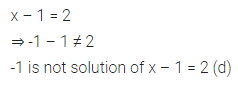Question 8.
The value of p for which the expressions p – 13 and 2p + 1 become equal is
(a) 0
(b) 14
(c) -14
(d) 5
Solution:Question 9.
The equation which cannot be solved in integers is
(a) 5x – 3 = -18
(b) 3y – 5 = y – 1
(c) 3p + 8 = 3 + p
(d) 9z + 8 = 4z – 7
Solution: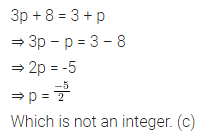Question 10.
The solution of which of the following equations is neither an integer nor a fraction?
(a) 2x + 5 = 1
(b) 3x – 7 = 0
(c) 5x – 7 = x + 1
(d) 4x + 7 = x + 2
Solution: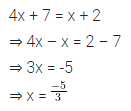Question 11.
If the sum of two consecutive even numbers is 54, then the smaller number is
(a) 25
(b) 26
(c) 27
(d) 28
Solution:Question 12.
If the sum of two consecutive odd numbers is 28, then the bigger number is
(a) 19
(b) 17
(c) 15
(d) 13
Solution:Question 13.
If 5 added to thrice an integer is -7, then the integer is
(a) -6
(b) -5
(c) -4
(d) 4
Solution: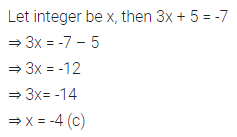Question 14.
If the length of a rectangle is twice its breadth and its perimeter is 120 m, then its length is
(a) 20 m
(b) 30 m
(c) 40 m
(d) 60 m
Solution:Question 15.
If the difference of two complementary angles is 10°, then the smaller angle is
(a) 40°
(b) 50°
(c) 45°
(d) 35°
Solution:Question 16.
If the difference of two supplementary angles is 30°, then the larger angle is
(a) 60°
(b) 75°
(c) 90°
(d) 105°
Solution:Question 17.
If x ∈ W, the solution set of the inequation -2 ≤ x < 3 is
(a) {-2, -1,0, 1, 2}
(b) {-1, 0, 1, 2, 3}
(c) {0, 1, 2, 3}
(d) (0, 1, 2}
Solution: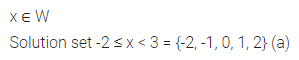Value Based Questions

Question 1.
On his 13th birthday, a boy decided to distribute blankets to the poor people instead of giving a party to his friends. Half of the blankets he distributed in an old age home, three fourths of the remaining in an orphanage and rest 20 were distributed to the roadside beggars. Find the number of blankets he had. What values are being promoted?
Solution: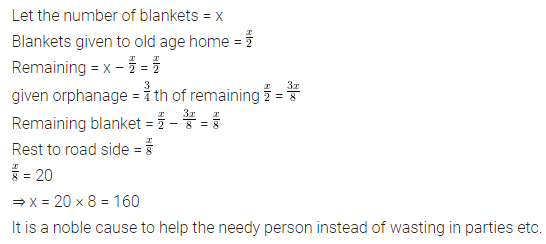Higher Order Thinking Skills (HOTS)

Question 1.
Two persons start moving from two points A and B in opposite directions towards each other. One person start moving from A at a speed of 4 km/h and meets the other person coming from B after 6 hours. If the distance between A and B is 42 km, find the speed of the other person.
Solution:Question 2.
There are some benches in the classroom. If 4 students sit on each bench then 3 benches remain empty and if 3 students sit on each bench then 3 students remain standing. Find the number of students in the class.
Solution: